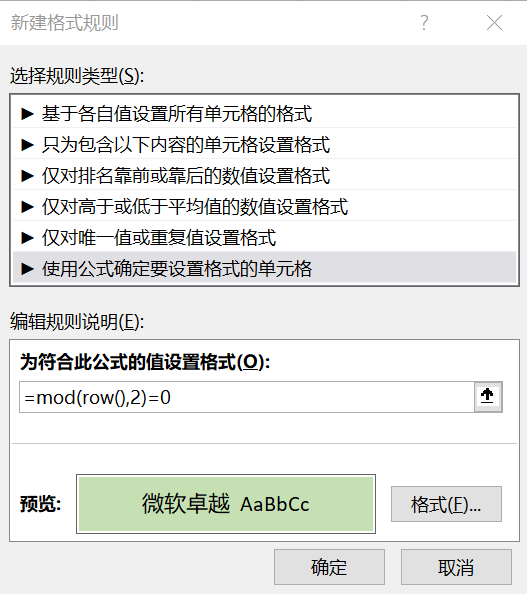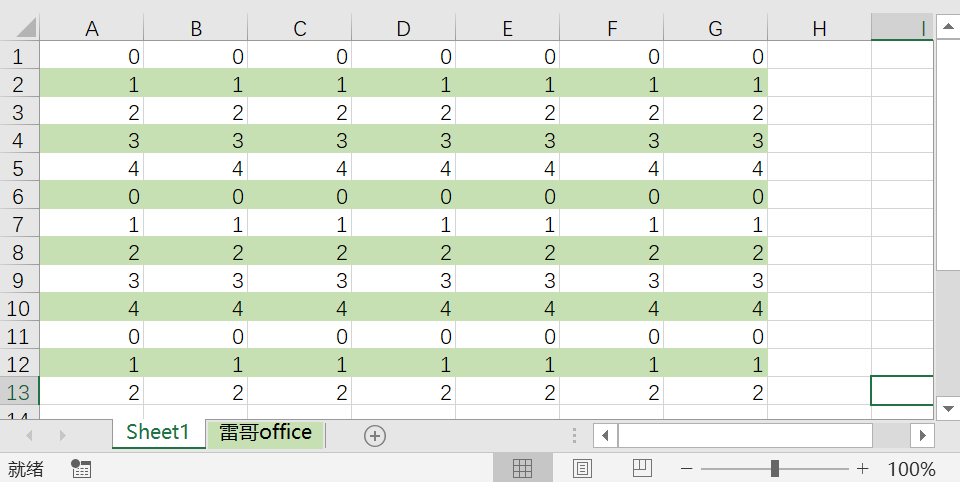01

# 函数基本用法

=MOD ( 参数1, 参数2 )
=参数1  –  参数2 *INT( 参数1 /参数2

=MOD(4,3) , 结果为1
=MOD(4,2) , 结果为0

02

# 深度理解MOD

① MOD中的两个参数允许为小数
=MOD(5,3.1), 结果为1.9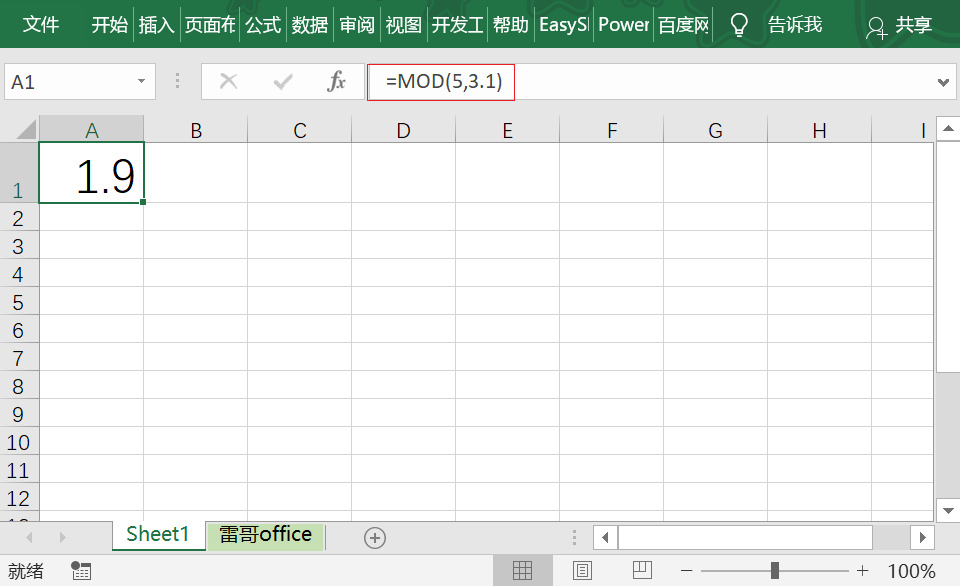② MOD的正负数跟参数二保持一致

=MOD(5,-3) 结果为 -1
解释：=参数1  –  参数2 *INT( 参数1 /参数2）= 5 – （-3）*INT(5/(-3))=5-(-3)*(-2)=-1

=MOD(-5,3) 结果为 1
解释：=参数1  –  参数2 *INT( 参数1 /参数2）= -5 – 3*INT(-5/3)=-5-3*(-2)=1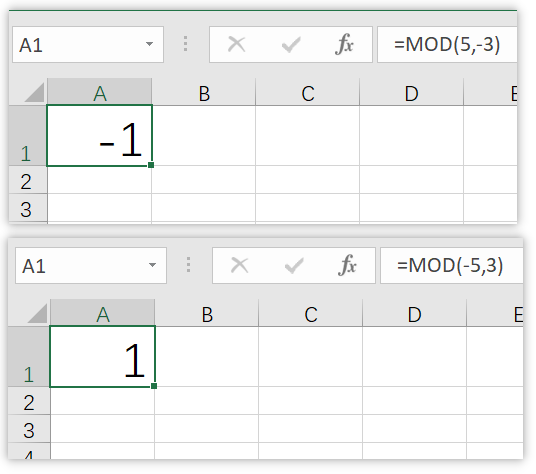03

# MOD应用案例

① 生成循环数据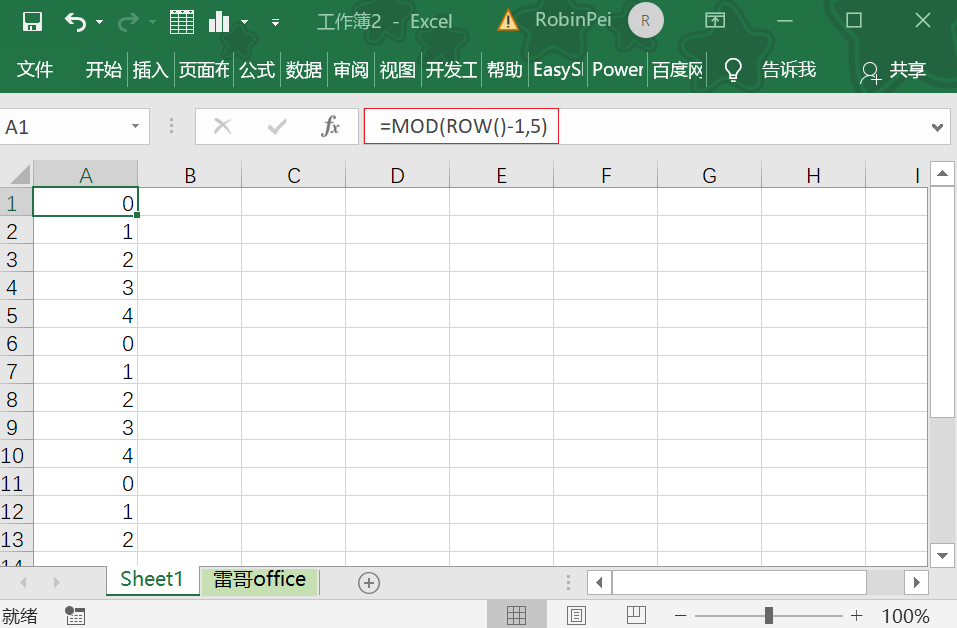② 隔行添加数据背景颜色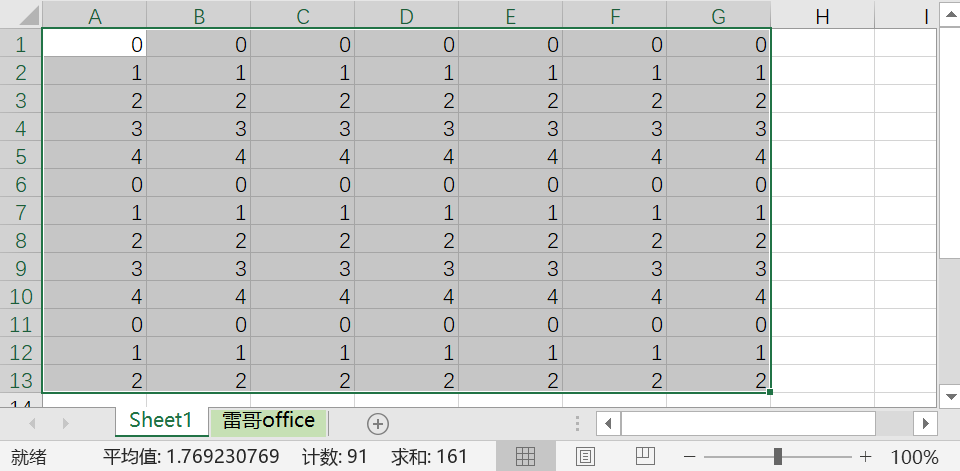step1：选中数据，单击【条件格式】，新建规则
step2：选择使用公式确定要设置格式的单元格，输入公式 =mod(row(),2)=0